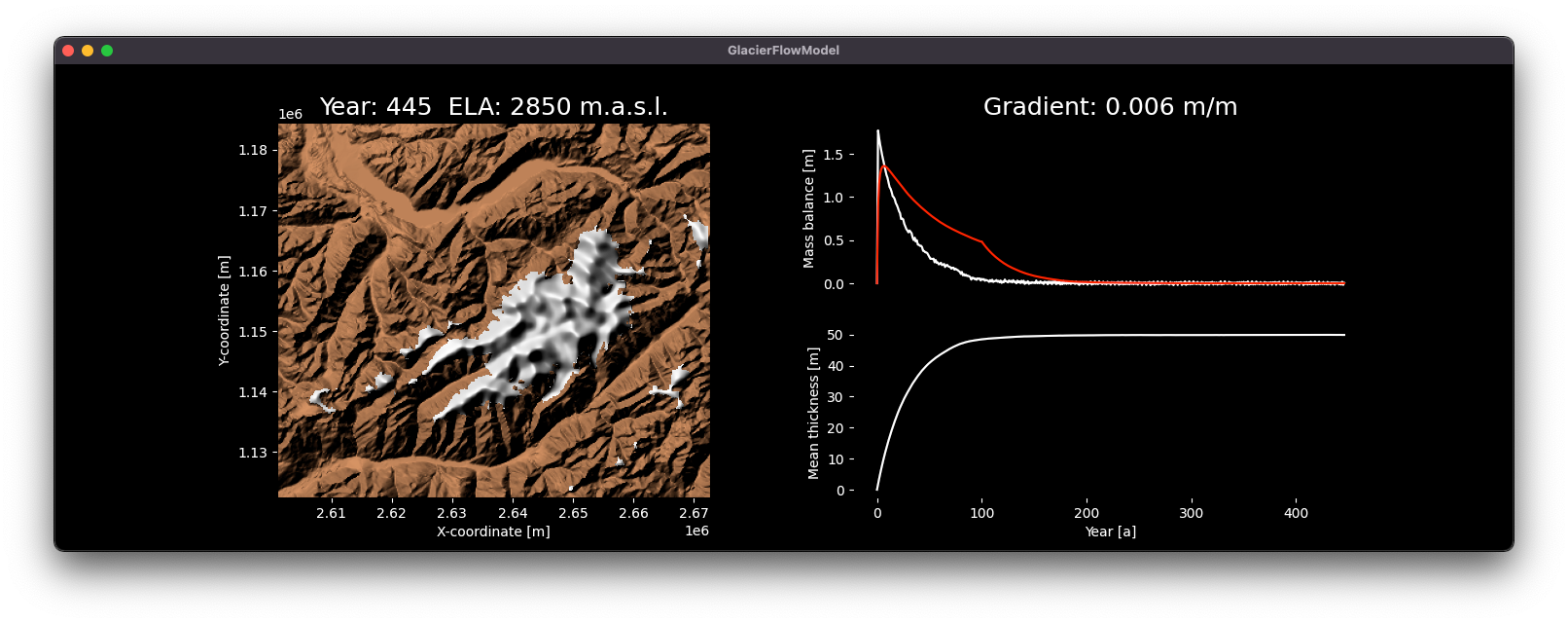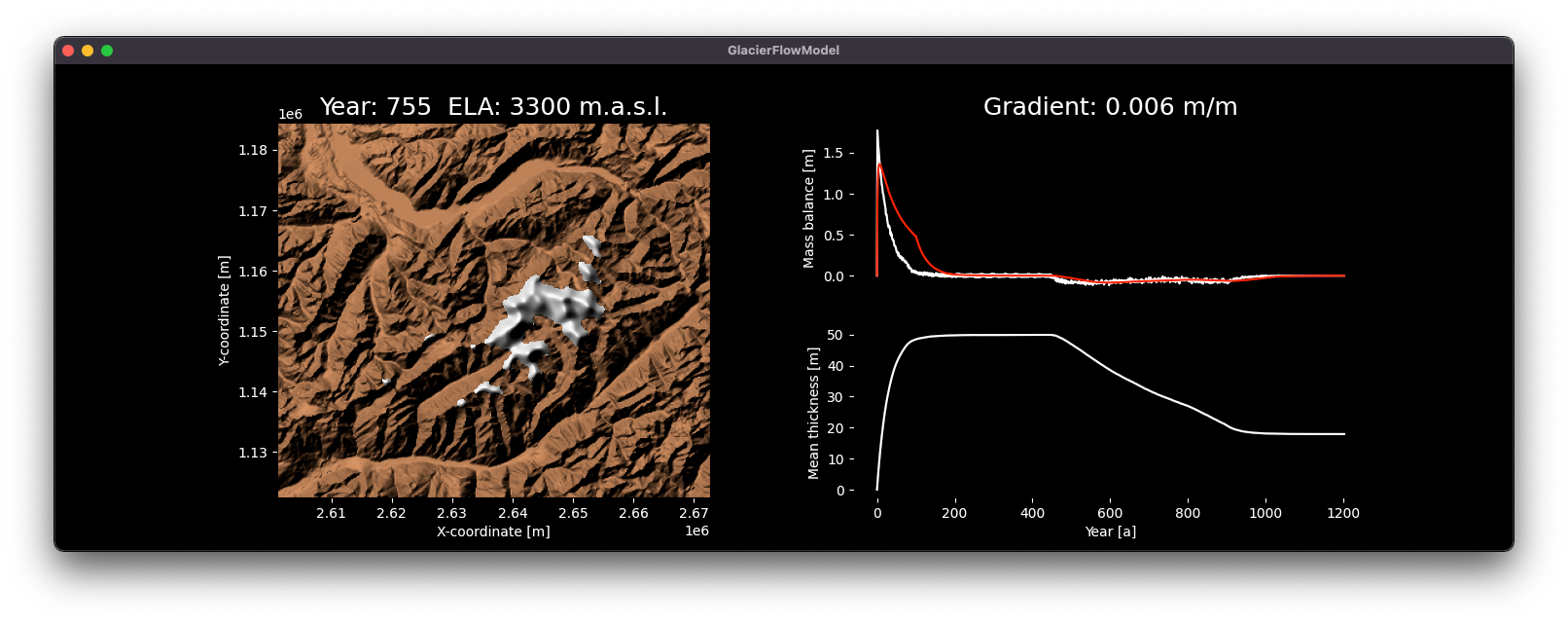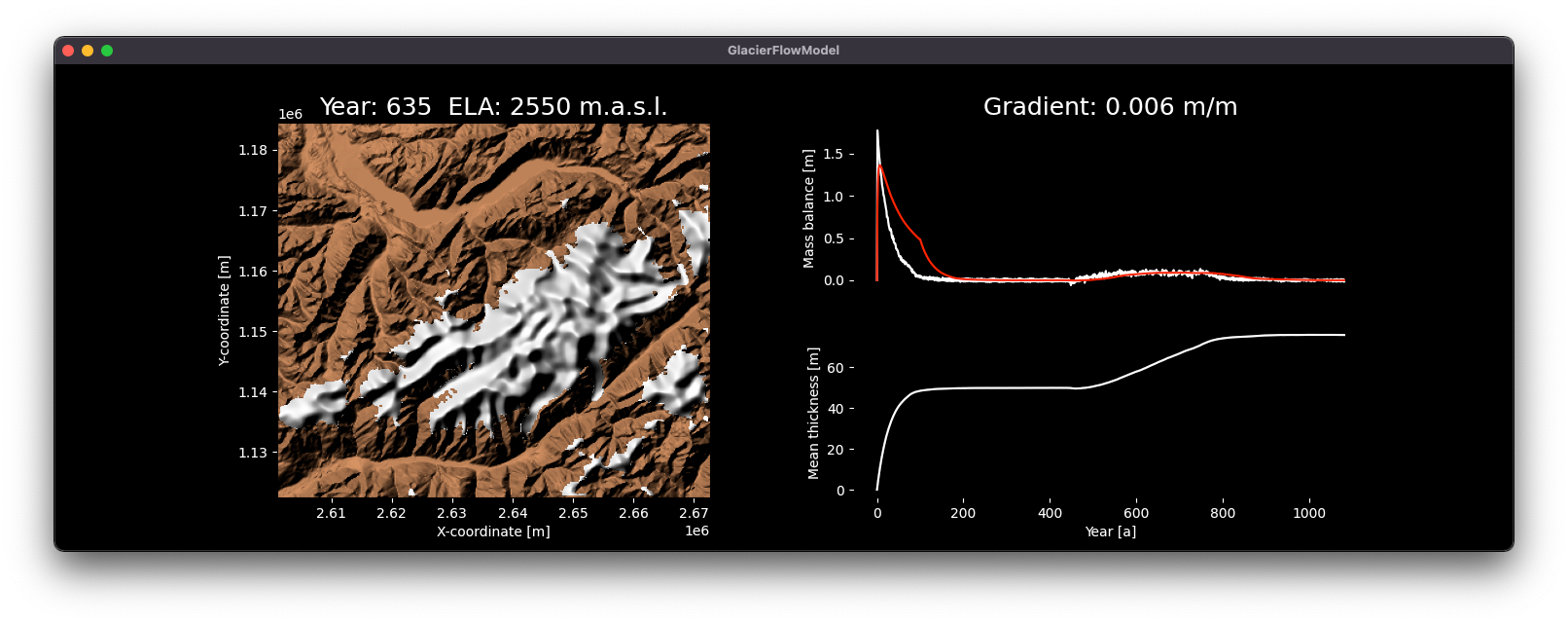# glacier-flow-model Release 0.1.2

Modeling glaciers flow, grounded on the glaciers mass balance and a digital elevation model (DEM).

Keywords
digital-elevation-model, geographical-information-system, geospatial, glacier, glaciers-flow, gradient, mass-balance, modeling
MIT
Install
``` pip install glacier-flow-model==0.1.2 ```

### Documentation## Glacier flow modelModeling glaciers on a digital elevation model (DEM) based on the mass balance of the glaciers and the D8 flow algorithm applied to the ice.

The modeling is based on the linear relationship between altitude and mass balance, the so-called mass balance gradient. For alpine glaciers this gradient is about 0.006 m/m. Continental glaciers tend to be at 0.003 and maritime glaciers at 0.01 m/m. The alpine gradient is set by default in the model. To model the glaciers, annual steps are calculated. First the mass balance (accumulation and ablation) for the area is added to the glacier layer and in a second step the glacier flow is simulated by using the D8 technique, which is known for modeling surface water flows over the terrain. In order to avoid pure convergence of the flow, a random impulse ("nudging") is added to the flow. Then the surface of the glaciers is slightly smoothed. The simulation stops when the observed difference in mass balance for a smoothed curve (n=-100) is less than 0.0001 m.

### Getting started

The glacier-flow-model package depends on GDAL, which needs to be installed on the system.

Get the stable release of the package from pypi:

`pip install glacier-flow-model`

#### Example data

The package includes an example DEM from swisstopo. It covers a smaller extent around the Aletsch glacial arena in Switzerland with a raster cell resolution of 200m.

```from glacier_flow_model import PkgDataAccess
pkg = PkgDataAccess()

#### Usage

To set up a glacier flow model, a path to a DEM in the GeoTiff (`.tif` or `.asc`) file format has to passed to the model class constructor. By default the mass balance parameters for alpine glaciers in the year 2000 are set. Keep the input file size small, otherwise the model may be slowed down remarkably:

```from glacier_flow_model import GlacierFlowModel, PkgDataAccess
pkg = PkgDataAccess()
gfm = GlacierFlowModel(pkg.locate_dem())```

After initialization, the model needs to accumulate the initial ice mass until it reaches a steady state, call the `reach_steady_state` method to do so:

`gfm.reach_steady_state()`When the model is in a steady state, a temperature change of the climate can be simulated. Simply use the `simulate` method with a positive or negative temperature change in degrees. The model changes the temperature gradually and simulates years until it reaches a steady state again.

Heating 4.5°C after initial steady state:

`gfm.simulate(4.5)`Cooling -1°C after initial steady state:

`gfm.simulate(-1)`Check out the video of the scenario simulation in the Aletsch glacial arena in Switzerland

### Limitations

The model has some limitations that need to be considered:

• The flow velocity of the ice per year is limited by the resolution of the grid cells. Therefore, a too high resolution should not be chosen for the simulation.
• The modeling of ice flow is done with D8, a technique for modeling surface flow in hydrology. Water behaves fundamentally different from ice, which is neglected by the model (e.g. influence of crevasses).
• No distinction is made between snow and ice. The density of the snow or ice mass is also neglected in the vertical column.